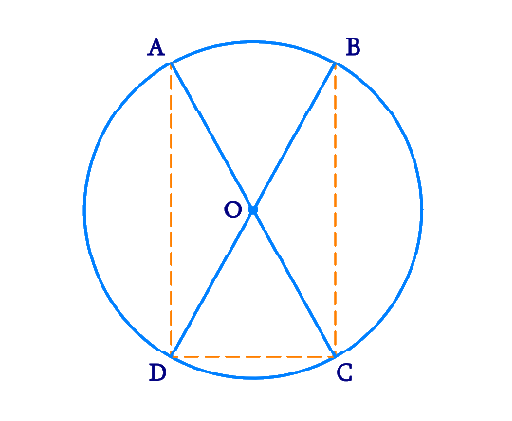# Ex.10.6 Q7 Circles Solution - NCERT Maths Class 9

Go back to  'Ex.10.6'

## Question

\begin {align}{AC}\end {align} and \begin {align} {BD}\end {align} are chords of a circle which bisect each other. Prove that

(i) \begin {align} {AC}\end {align} and \begin {align} {BD}\end {align} are diameters,

(ii) \begin {align} {ABCD}\end {align} is a rectangle.

Video Solution
Circles
Ex 10.6 | Question 7

## Text Solution

What is known?

\begin {align} {AC}\end {align} and \begin {align}{BD}\end {align} are $$2$$ chords of a circle which bisect each other.

What is unknown?

Proof that \begin {align} {AC}\end {align} and \begin {align} {BD}\end {align} are diameters and \begin {align} {ABCD}\end {align} is a rectangle.

Reasoning:

• A quadrilateral \begin {align} {ABCD}\end {align} is called cyclic if all the four vertices of it lie on a circle.
• The sum of either pair of opposite angles of a cyclic quadrilateral is $$180^{\circ}.$$
• Opposite angles in a parallelogram are equal.
• Using Side-Angle-Side (SAS criteria) and Corresponding parts of congruent triangles (CPCT) we prove the statement.

Steps:Let \begin {align} {AC}\end {align} and \begin {align} {BD}\end {align} be $$2$$ chords intersecting at \begin {align} {O.}\end {align}

In \begin {align} \Delta {AOB}\end {align} and \begin {align} \Delta {COD,}\end {align}

\begin{align}{OA}&={OC} \quad (\text { Given }) \\ {OB}&={OD} \quad \text { (Given) } \\ \angle {AOB}&=\angle {COD} \\ \text {(Vertically } & \text{opposite} \text{ angles }) \end{align}

$$∴ \Delta {AOB} \cong \Delta {COD}$$ (SAS congruence rule)

$${AB} ={CD}$$ (By CPCT)

Similarly, it can be proved that \begin{align} \Delta {AOD} \cong \Delta {COB} \end{align}

\begin{align} ∴ {AD}={CB} \qquad (\text{By CPCT}) \end{align}

Since in quadrilateral \begin{align} {ABCD,} \end{align} opposite sides are equal in length, \begin{align} {ABCD} \end{align} is a parallelogram.

We know that opposite angles of a parallelogram are equal.

\begin{align} ∴ \angle {A}=\angle {C} \end{align}

However,

\begin{align} \angle {A} \!+ \! \angle {C} \!&=\!180^{\circ} \\{(ABCD} &\text { is a cyclic } \text{ quadrilateral) } \\\angle {A}+\angle {A} &=180^{\circ} \\ 2 \angle {A}&=180^{\circ} \\ ∴ \angle {A}&=90^{\circ}\end{align}

As \begin{align}{ABCD}\end{align} is a parallelogram and one of its interior angles is $$90^{\circ,}$$ therefore, it is a rectangle.

\begin{align}\angle {A}\end{align} is the angle subtended by chord \begin{align}\angle {BD.}\end{align}

And as .\begin{align}\angle {A} = 90^{\circ}, \end{align} therefore, \begin{align} {BD}\end{align} should be the diameter of the circle. Similarly, \begin{align} {AC}\end{align} is the diameter of the circle.

Video Solution
Circles
Ex 10.6 | Question 7

Learn from the best math teachers and top your exams

• Live one on one classroom and doubt clearing
• Practice worksheets in and after class for conceptual clarity
• Personalized curriculum to keep up with school